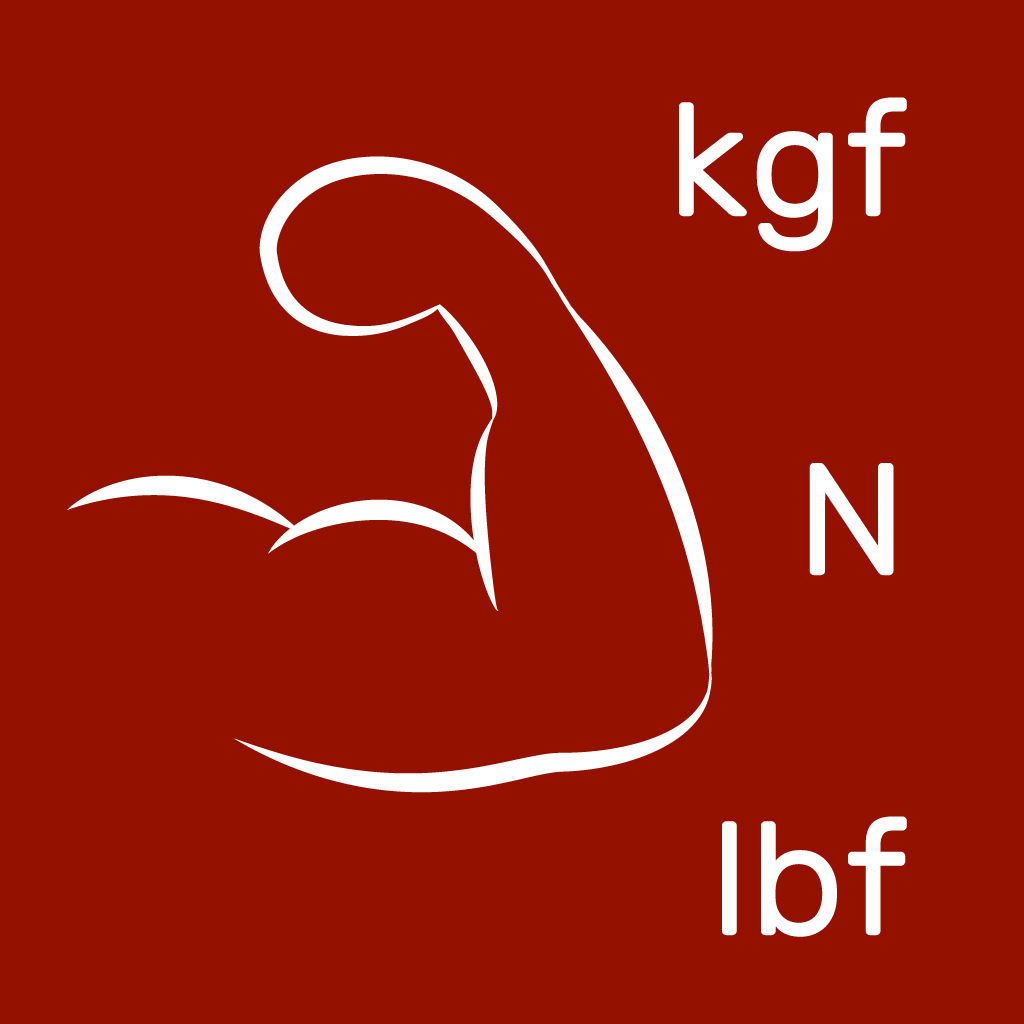# Force Converter

Force Converter is the fast force converter to convert for Newton (N), kilonewton (kN), ton-force (tf), kilogram-force (kgf), pound-force (lbf).

You can use it if you physicist, builder, engineer or another cool man.

Convert forces quickly and as you want.

View on App Store